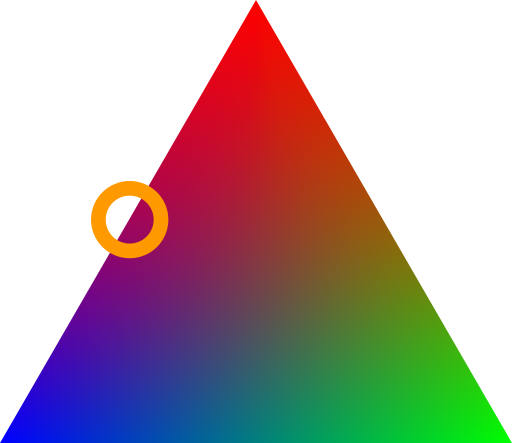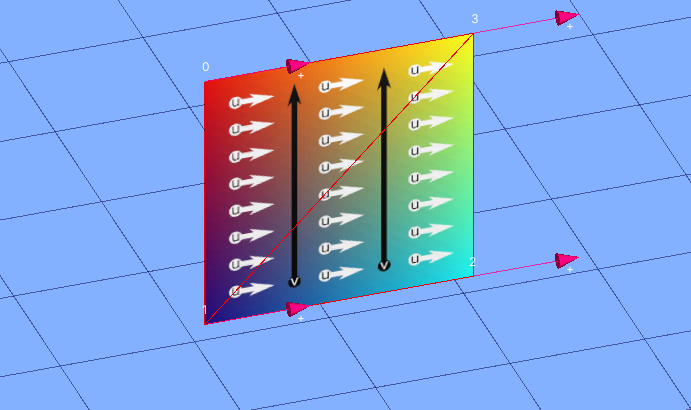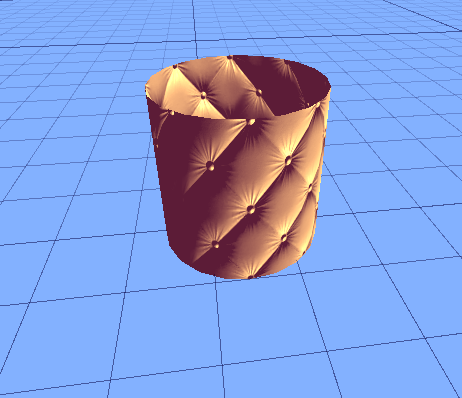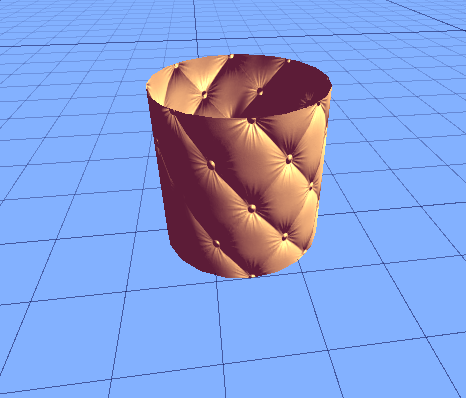# Procedural Meshes in Unity: Normals and Tangents

In previous posts, we looked at general mesh generation in Unity, and the issues that arise in generating meshes for lines. In this post, I explain normals and tangents, which you need to calculate for meshes you generate in certain situations.

## Normals

Normals are vectors perpendicular to the mesh. Mathematically there are two such normals (in opposite directions), however, in mesh rendering only one side of a triangle is visible, and by convention, only one of the two normals is used — the one pointing away from the visible face.

Normals are used in lighting calculations, and for a mesh we can specify a normal for each vertex.

Roughly, the orientation of a triangle determines how much light it will reflect (it also depends on the angle of the light and camera).

We can quite easily determine the normals for a triangle by taking the cross product of the vectors made from any two sides.

And indeed, Unity provides a convenience method that does this, and we have been using it for the normal calculations on our 2D meshes. In this case, the three normals will be the same.

But they need not be the same, and when they are different, behind the scene the system will interpolate them so that for each position on the triangle, there will be a normal calculated based on how far the point is to the vertices of the triangle. If we represent the normals as colors, we can get a picture of how this will look:Each triangle has three normals associated with it — the three normals given for the three vertices. Normals are interpolated over the triangle, the same as UVs. For example, The normal at the center of the orange ring would be pointing halfway between the normals of the red and blue vertices.

As you can see, the colors are smooth, and indeed so are the normals. The effect is that the triangle is rendered as if its normals are changing smoothly across the triangle, making it look curved! This is the reason you cannot see where the triangles are in a sphere or cylinder.

When two triangles have a vertex in the same position, we have a choice whether to share it (so there is just one instance in our vertex list), or not (so there are two copies of the vertex in our vertex list). Which one you choose is determined by whether you need one of the other lists (UVs, normals, or tangents) to have separate data for vertices of different triangles.

In the case of normals, if the normals are the same for all vertices at a point, the surface will look smooth. If we want flat surfaces instead, we need to have vertices separate. (Of course, we may need different things at different places, so our decision need not be made uniform over the entire mesh). We already looked at how to calculate correct normals for flat surfaces. How do we calculate normals for smooth objects?

There are two ways we can go about it:

1. Since the shape is generated procedurally, we (probably) have a way to calculate the correct normal directly. This is often the case with mathematical objects (spheres, cylinders, etc.). In fact, if we have a parameterization of the surface, we there is a general purpose formula for the normal.

2. If it is not possible to calculate a normal directly, we can compute flat normals for each triangle a vertex position lies in, and average them out. Of course, averaging directions need some care.

If the surface is “fairly smooth”, you can probably get away with a straight linear average and normalize:

normal = ((normal_1 + normal_2 + ... + normal_i) / i).normalize;

This solution has some problems:

• It is not correct if all the normals do not point into the same halfplane. When this happens there is a problem with the mesh in any case (something is wrong, or the surface is not “fairly smooth”).
• It is not correct if normals all lie in a straight line. As before, either something is wrong, or the curve is not “fairly smooth”.
• You may run into issues if your triangle distribution is unequal. For example, suppose we have a sphere, and at the pole we have double the number of triangles on the left as on the right. This will skew the normal. In such cases, you may want to weigh the normals in the average, perhaps by the angle they make around the vertex.

For many every-day purposes you should not have to worry about these issues.

## Tangents

Tangents are usually used by bump shaders, and like normal maps convey information about the surface’s orientation at a vertex. Tangents are parallel to the surface at the vertex (that is, perpendicular to the normals), and lie in the U direction of the texture at that vertex. A tangents is represented as a Vector4; the first three components is the direction of the tangent, and the last component is 1 or -1, used to flip the tangents.Here pink arrows show the direction of the tangents for a simple quad. There are four, one for each vertex. Notice they point in the same direction as the U direction, shown by white arrows in the texture.Tangents are flipped. Notice that the lighting of the bumps has changed, but the overall lighting direction is the same.Tangents in the right direction. (The only way to tell this is the correct version, in this case, is to look at the actual light direction. I usually compare the lighting of the mesh with that of a sphere.)

Normally, you would use your knowledge of the shape directly to calculate the tangent, although technically you could use calculations from UVs and the vertices to construct a tangent.

It also seems that Unity calculates decent tangents if you don’t provide any, although I have no idea whether this is robust when the meshes are more complicated.

## Example: Open Ended Cylinder

In this example, we will be generating a mesh for a open-ended double sided cylinder with smooth normals. Even though the mesh is double sided, we will only do calculations for the outside. The inside will be automatically be generated from a special method (given at the end).

Here is a simplified schematic of the vertex setup we will use. Notice that two vertices are duplicated; this is so we can have separate UVs so that triangles 7,8,1 and 7, 6, 1 will be textured correctly. Other vertexes between neighboring triangles are shared, since we are making a smooth mesh.

We will have three parameters:

• n, the number of quads that make up the cylinder. In the diagram above, n is 6.
• The radius of the cylinder.
• The height of the cylinder.

Vertices. We already saw how to calculate vertices for a circle (but this time we duplicate the first and last vertex). We can use this for the green vertices; for the red vertices we simply replicate the green ones and adjust their y coordinate to the height of the cylinder.

There are 2n + 2 vertices in total.

Triangles.

UVs. For the UVs, we simply divide the rectangle into n segments. The bottom UVs are given by (i / n, 0), and the top UVs by (i / n, 1), where i ranges from 0 to n.

Normals. Normals are parallel to the XZ plane, and point away from the central axis of the cylinder. Below the direction of the normals and tangents are shown from the top.

The normals in this case is easily calculated from the vertices:

normal[i] = vertex[i];
normal[i].y = 0; //ignore height
normal[i].Normalize();

Tangents. The tangents are also easily calculated from the vertices, or even easier from the normals.

tangent[i] = normal[i].PerpXZ();

## Tips

Remember:

• You cannot share the first and last vertex in loops if the UVs are not the same.
• You cannot share vertices if you want hard edges.
• The triangle count is the same regardless of whether you share some vertices or not.

Test all debugging techniques with a simple quad first. If you use any debugging technique for the first time, make sure to test it on a quad first to see if you get the results you expect. This will eliminate a common source of confusion: incorrect debug code or assets.

Use a generic way to build double sided meshes. Remember triangles are visible from only one side. If you want to see the other side too, you need to replicate all lists, and flip normals, and flip triangles. The MeshData class class below can be used to combine meshes, as well as make them double sided.

Render arrows in normal and tangent directions. For the latter, you may also want to use a special texture that clearly marks the positive U direction with arrows; you should then compare this with the tangents and make sure they match. Also, render a plus or minus depending on whether the w component of the tangent is +1 or -1. Notice that the gradient texture below also allows us to see UV seems.

Use a special shader and material to visualize normals as colors. In this case, put a sphere with the same debug material in your scene and compare the colors of your mesh with those on the sphere. It is important whether your debug shader uses world space or local space. If the latter, the sphere needs to be in the same orientation as the mesh; otherwise it does not matter. The code for such as shader is given below. The vector components range between -1 and 1, but colors go from 0 to one, so we need to map vectors to colors. There are two basic ways:

• Scale and shift. 0.5*x + 0.5. This method allows you to distinguish opposite vectors, but can be hard to read.
• Take the absolute value. abs(x). This is easier to read (the redder, the more in the +/- x direction something lies). However, it cannot distinguish between opposite vectors, and having normals in the opposite direction is a common bug.

Build a special debug light rig. This can be used instead of the shader described above. Either use three or six lights. In both setups, we have red, green, and blue lights along the directions of the axes, but in the second we one in each of the two directions. This setup can be more useful if you also need to see the #D shape of the object (and is quicker to implement if you don’t have a shader at hand. If you use this method, remember to remove other light sources, including ambient light, to avoid confusion.

Show the bounding box info. This will help you spot when points are too far apart (and far from the camera) or too close together to make the mesh visible. Note It is not enough to render the bounding box, as the bounding box too will be invisible if it is too big or small.

## Code

Shader "Debug/Normals"
{
Properties
{
[Toggle(USE_WORLD_SPACE)]
_UseWorldSpace("Use World Space", Float) = 0

[Toggle(USE_STANDARD_COLOR_MAP)]
_UseStandardColorMap("Use Standard Color Map", Float) = 0
}

{

Pass
{
CGPROGRAM
#pragma vertex vert
#pragma fragment frag
// include file that contains UnityObjectToWorldNormal helper function

#include "UnityCG.cginc"

struct v2f
{
// we'll output world space normal as one of regular ("texcoord") interpolators
half3 normal : TEXCOORD0;
float4 pos : SV_POSITION;
};

// vertex shader: takes object space normal as input too
v2f vert(float4 vertex : POSITION, float3 normal : NORMAL)
{
v2f o;
o.pos = UnityObjectToClipPos(vertex);

#ifdef USE_WORLD_SPACE
// UnityCG.cginc file contains function to transform
// normal from object to world space, use that
o.normal = UnityObjectToWorldNormal(normal);
#else
o.normal = normal;
#endif

//o.normal = normal;// UnityObjectToWorldNormal(normal);

return o;
}

fixed4 frag(v2f i) : SV_Target
{
fixed4 c = 0;
#ifdef USE_STANDARD_COLOR_MAP
c.rgb = i.normal * 0.5 + 0.5;
#else
c.rgb = abs(i.normal);// *0.5 + 0.5;
#endif
return c;
}
ENDCG
}
}
}

### MeshData

public class MeshData
{
public List<Vector3> vertices;
public List<int> triangles;
public List<Vector2> uvs;
public List<Vector3> normals;
public List<Vector4> tangents;
public static MeshData Combine(List<MeshData> meshes)
{
MeshData combinedMesh = new MeshData
{
vertices = new List<Vector3>(),
triangles = new List<int>(),
uvs = new List<Vector2>(),
normals = new List<Vector3>(),
tangents = new List<Vector4>()
};

int vertexIndexOffset = 0;

foreach(var mesh in meshes)
{

if(mesh.uvs != null)
{
}

if(mesh.normals != null)
{
}

if(mesh.tangents != null)
{
}

vertexIndexOffset += mesh.vertices.Count;
}

return combinedMesh;
}

public MeshData Flip()
{
return new MeshData
{
vertices = vertices,
uvs = uvs,
normals = MeshBuilderUtils.FlipNormals(normals),
triangles = MeshBuilderUtils.FlipTriangles(triangles),
tangents = tangents
};
}

//Does not check if already double sided
public MeshData GetDoubleSided()
{
var meshes = new List<MeshData>
{
this,
this.Flip()
};

return Combine(meshes);
}
}

### Basic Mesh Builder

using Gamelogic.Extensions;
using System;
using System.Collections.Generic;
using System.Linq;
using UnityEditor;
using UnityEngine;

public enum MeshType{
XYZ,
XY,
XZ
}

[Serializable]
public class DebugInfo
{
public int vertexCount = 0;

public int triangleIndexCount = 0;

public int normalCount = 0;

public int uvCount = 0;

public Bounds bounds;
}

[RequireComponent(typeof(MeshFilter))]
public class MeshBuilder: MonoBehaviour
{
private readonly static Color DebugSphereColor = new Color(1, 0.25f, 0);
private readonly static Color DebugNormalColor = new Color(1, 1, 0);
private readonly static Color DebugTangentColor = new Color(1, 0, .5f);
private const float SmallestValidTriangleArea = 0.01f;

[SerializeField]
private DebugInfo debugInfo = new DebugInfo();

[SerializeField]
private bool drawDebugVertices = false;

[SerializeField]

[SerializeField]
private bool drawDebugLabels = false;

[SerializeField]
private bool printDebugInfo = false;

[SerializeField]
private bool drawDebugNormals = false;

[SerializeField]
private bool drawDebugTangents = false;

[SerializeField]
private MeshType meshType = MeshType.XYZ;

//Use property instead to ensure initialization
private MeshFilter meshFilter;

//We keep this as a variable so we can draw debug Info
private List<Vector3> vertices;

//Same
private List<Vector3> normals;

//Same
private List<Vector4> tangents;

private GUIStyle vertexLabelStyle;

[HideInInspector]
[SerializeField]
protected GeometryDebug meshDebug;

protected MeshFilter MeshFilter
{
get
{
if (meshFilter == null)
{
meshFilter = GetComponent<MeshFilter>();
//cannot be null since MeshFilter is required.
}

return meshFilter;
}
}

public void UpdateMesh()
{
if (meshDebug == null)
{
meshDebug = new GeometryDebug();
}

meshDebug.Clear();

DestroyOldMesh();
Preprocess();

var mesh = new Mesh();

vertices = CalculateVertices();
debugInfo.vertexCount = vertices.Count;

DebugLog("Vertices", debugInfo.vertexCount);
mesh.SetVertices(vertices);

var triangles = CalculateTriangles();
debugInfo.triangleIndexCount = triangles.Count;

DebugLog("Triangles", debugInfo.triangleIndexCount);
mesh.SetTriangles(triangles, 0);

//We set triangles before doing this test so that the number of
//vertex indices and their range can be checked before we execute
//the code below, that will
ValidateTriangleAreas(triangles);

var uvs = CalculateUvs(vertices);

if (uvs == null)
{
//can be null if subclass
debugInfo.uvCount = -1;
DebugLog("Uvs", null);
}
else
{
debugInfo.uvCount = uvs.Count;
DebugLog("Uvs", debugInfo.uvCount);
mesh.SetUVs(0, uvs);
}

normals = CalculateNormals();

if (normals == null)
{
//can be null if subclass does override default normals
DebugLog("Normals", null);
mesh.RecalculateNormals();
normals = new List<Vector3>();
mesh.GetNormals(normals);

debugInfo.normalCount = normals.Count;
}
else
{
debugInfo.normalCount = normals.Count;
DebugLog("Normals", debugInfo.normalCount);
mesh.SetNormals(normals);
}

tangents = CalculateTangents();

if (tangents == null)
{
tangents = new List<Vector4>();
mesh.GetTangents(tangents);
}
else
{
mesh.SetTangents(tangents);
}

mesh.RecalculateBounds();
debugInfo.bounds = mesh.bounds;
meshFilter.sharedMesh = mesh;
}

virtual protected List<Vector4> CalculateTangents()
{
var tangent = new Vector4(1, 0, 0, 1);
return new List<Vector4>
{
tangent,
tangent,
tangent,
tangent
};
}

private void ValidateTriangleAreas(List<int> triangles)
{
for (int i = 0; i < triangles.Count / 3; i++)
{
int vertexIndexA = triangles[3 * i];
int vertexIndexB = triangles[3 * i + 1];
int vertexIndexC = triangles[3 * i + 2];
var vertexA = vertices[vertexIndexA];
var vertexB = vertices[vertexIndexB];
var vertexC = vertices[vertexIndexC];

float area = MeshBuilderUtils.AreaOfTriangle(vertexB - vertexA, vertexC - vertexA);

if (area < SmallestValidTriangleArea)
{
Debug.LogWarning(
string.Format(
"Triangle is too small. <{0}, {1}, {2}>, <{3}, {4}, {5}>",
vertexIndexA, vertexIndexB, vertexIndexC, vertexA, vertexB, vertexC
));
}
}
}

public void OnDrawGizmos()
{
if (vertices == null) return; //can happen if no mesh was ever created.

//if(vertexLabelStyle == null)
{
vertexLabelStyle = new GUIStyle();
vertexLabelStyle.normal.textColor = Color.white;
vertexLabelStyle.alignment = TextAnchor.MiddleCenter;
}

if(drawDebugVertices)
{

for (int i = 0; i < vertices.Count; i++)
{
var vertex = vertices[i];
var spherePosition = transform.TransformPoint(vertex);

}

if (meshDebug != null)
{
meshDebug.Draw(transform, meshType);
}
}

if (drawDebugNormals)
{
for (int i = 0; i < normals.Count; i++)
{
var vertex = vertices[i];
var transformedPosition = transform.TransformPoint(vertex);

var normal = normals[i];
var transformedNormal = transform.TransformDirection(normal);
float length = 2 * transform.lossyScale.magnitude * debugSphereRadius;

GeometryDebug.DrawArrow(transformedPosition, transformedNormal, length, DebugNormalColor);
}
}

if (drawDebugTangents)
{
for (int i = 0; i < tangents.Count; i++)
{
var vertex = vertices[i];
var transformedPosition = transform.TransformPoint(vertex);
var normal = tangents[i];
var transformedNormal = transform.TransformDirection(normal);
float length = 2 * transform.lossyScale.magnitude * debugSphereRadius;

var label = (normal.w > 0) ? "+" : ((normal.w < 0) ? "-" : null);
GeometryDebug.DrawArrow(transformedPosition, transformedNormal, length, DebugTangentColor, label);
}
}

if (drawDebugLabels)
{
for (int i = 0; i < vertices.Count; i++)
{
var vertex = vertices[i];

var spherePosition = transform.TransformPoint(vertex);

Handles.Label(spherePosition + Vector3.up * radius / 2, i.ToString(), vertexLabelStyle);
}
}
}

virtual protected List<Vector3> CalculateVertices()
{
}

virtual protected List<Vector2> CalculateUvs(List<Vector3> vertices)
{
return MeshBuilderUtils.GetStandardUvsXY(vertices, true, true);
}

virtual protected void Preprocess() { }

virtual protected List<int> CalculateTriangles()
{
return new List<int>
{
0, 3, 1,
1, 3, 2
};
}

virtual protected List<Vector3> CalculateNormals()
{
return null;
}

protected void UpdateMeshTest()
{
UpdateMesh();
}

private void DestroyOldMesh()
{
if (MeshFilter.sharedMesh != null)
{
if (Application.isPlaying)
{
Destroy(MeshFilter.sharedMesh); //prevents memory leak
}
else
{
DestroyImmediate(MeshFilter.sharedMesh);
}
}
}

protected void DebugLog(string label, object message)
{
if (!printDebugInfo) return;

if (message == null)
{
DebugLog(label, "null");
}
else
{
Debug.Log(label + ": " + message.ToString(), this);
}
}

protected void DebugAddDotXY(Vector3 position, GLColor color)
{
}

public void DebugAddArrow(Vector3 position, Vector3 direction, GLColor color)
{
}

private void OnValidate()
{

if (MeshFilter.sharedMesh == null) return;

if (vertices == null)
{
vertices = MeshFilter.sharedMesh.vertices.ToList();

DebugLog("Vertices", "Refreshed from mesh.");
}

if(normals == null)
{
normals = MeshFilter.sharedMesh.normals.ToList();

DebugLog("Normals", "Refreshed from mesh.");
}

if(tangents == null)
{
tangents = MeshFilter.sharedMesh.tangents.ToList();

DebugLog("Tangents", "Refreshed from mesh.");
}
}
}


### Geometry Debug

public class GeometryDebug
{
public List<Vector3> dotPositions;
public List<GLColor> dotColors;
public List<Vector3> arrowPositions;
public List<Vector3> arrowDirections;
public List<GLColor> arrowColors;

public List<Color> colorMap = new List<Color>
{
Color255(255, 70, 70),
Color255(255, 150, 0),
Color255(255, 255, 60),
Color255(150, 220, 0),
Color255(25, 174, 255),
Color255(186, 0, 255),
Color255(255, 0, 193),
Color255(0, 0, 0),
Color255(255, 255, 255),
};

private static GUIStyle labelStyle;

public static GUIStyle LabelStyle {
get
{
if(labelStyle == null)
{
labelStyle = new GUIStyle
{
normal = new GUIStyleState { textColor = Color.white }
};
}

return labelStyle;
}
}

private static Color Color255(int r, int g, int b, int a = 255)
{
return new Color(r / 255f, g / 255f, b / 255f, a / 255f);
}

{
get; set;
} = 0.1f;

public GeometryDebug()
{
dotPositions = new List<Vector3>();
dotColors = new List<GLColor>();

arrowPositions = new List<Vector3>();
arrowDirections = new List<Vector3>();
arrowColors = new List<GLColor>();
}

public void Clear()
{
dotPositions.Clear();
dotColors.Clear();

arrowPositions.Clear();
arrowDirections.Clear();
arrowColors.Clear();
}

public void AddDotXY(Vector3 position, GLColor color)
{
}

public void AddArrow(Vector3 position, Vector3 direction, GLColor color)
{
}

public void Draw(Transform transform, MeshType meshType = MeshType.XYZ)
{
for(int i = 0; i < dotPositions.Count; i++)
{
var position = transform.TransformPoint(dotPositions[i]);
Color color = colorMap[(int)dotColors[i]];

}

for(int i = 0; i < arrowPositions.Count; i++)
{
var position = transform.TransformPoint(arrowPositions[i]);
var direction = transform.TransformDirection(arrowDirections[i]);
float size = transform.lossyScale.magnitude * 0.2f;

Color color = colorMap[(int)arrowColors[i]];

DrawArrow(position, direction, size, color);
}
}

public static void DrawArrow(Vector3 position, Vector3 direction, float size, Color color, string label = null)
{
Handles.color = color;
Handles.ArrowHandleCap(0, position, Quaternion.LookRotation(direction), size, EventType.Repaint);

if (label != null)
{
Handles.Label(position + size * direction, label, LabelStyle);
}
}

public static void DrawDot(Vector3 position, float radius, Color color, MeshType meshType)
{
switch (meshType)
{
case MeshType.XYZ:
Gizmos.color = color;
break;

case MeshType.XY:
Handles.color = color;
}